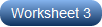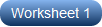# Solving Quadratic Equations Using the Formula Worksheets

Not all of us are born with exceptional math skills, but these can be built with practice. This batch of printable solving quadratic equations using the formula worksheets is a rare find to practice the easiest method of solving messy looking quadratic equations using the quadratic formula. Ensure that every step goes smoothly, and leads to answering quickly and intelligently, by simply identifying the coefficients a, b, and c from the quadratic equation, and substituting it in the formula x = (-b± √ (b2-4ac)) / 2a to find the roots. Using the formula is a never-fail method to solve quadratic equations, and memorizing the formula does the trick. What's even more laudable is the fact that there are no tedious steps to follow, just a plug and chug process. With our free worksheet, you will be well on the way to a great start.

Find the Roots | Quadratic Formula - Easy

Using the formula to solve the quadratic equation is just like waving a wand. With the equations presented in the standard form and involving only integers, identifying the coefficients a, b, and c, plugging them in the quadratic formula and solving is all that high school students need to do to find the roots.Find the Roots | Quadratic Formula - Moderate

As skill-building as they are engaging, these pdf worksheets on finding the roots of a quadratic equation using the formula help students go beyond the basics with decimal, fractional, and radical coefficients. Bring the equation to the standard form, plug the values in the formula, and chug the roots.Find the Roots | Substitution Method and Quadratic Formula

Prepare students to tackle tougher equations with this set of printable solving quadratic equations worksheets using the formula. Assign a variable to bring the equation that is in disguise to the standard form. Identify the coefficients, substitute and solve. Back-solve to find the value of the variable.Find the Roots | Equations Reducible to Quadratic Form

Notice an uptick in student engagement with this bundle of pdf worksheets with equations reducible to quadratics. Get high school students to reduce the polynomial equation to a quadratic and then plug the coefficients in the quadratic formula, and solve to find the roots of the quadratic equations.Related Worksheets